# RD Sharma Solutions Chapter 14 Coordinate Geometry Exercise 14.4 Class 10 Maths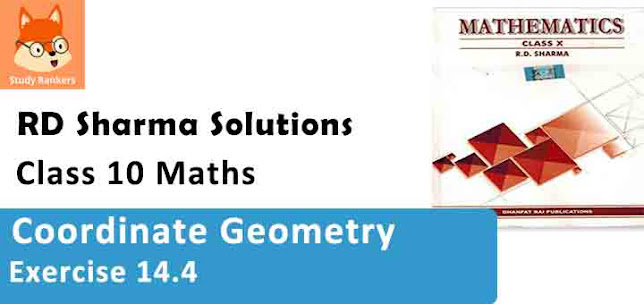Chapter Name RD Sharma Chapter 14 Coordinate Geometry Book Name RD Sharma Mathematics for Class 10 Other Exercises Exercise 14.1Exercise 14.2Exercise 14.3Exercise 14.4Exercise 14.5VSAQs and MCQs Related Study NCERT Solutions for Class 10 Maths

### Exercise 14.2 Solutions

1. Find the centroid of the triangle whose vertices are :

(i) (1, 4), (-1, -1), (3, -2)
(ii) (-2, 3), (2, -1), (4, 0)

Solution

(i) Coordinates of the centroid are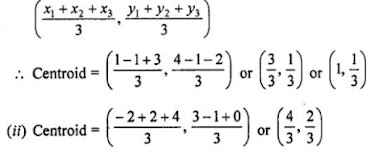2. Two vertices of a triangle are (1, 2), (3, 5) and its centroid is at the origin. Find the Co-ordinates of the third vertex.

Solution

Centroid of a triangle is O(0, 0) …(i)
Co-ordinates of two vertices of a ∆ABC are A (1, 2) and B (3, 5)
Let the third vertex be (x, y)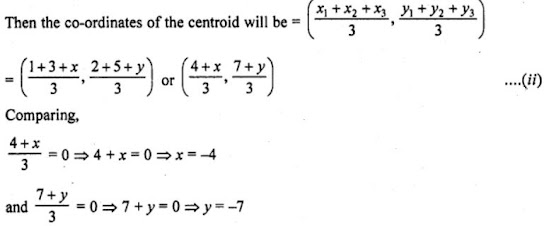The vertex is (-4, -7)

3. Find the third vertex of a triangle, if two of its vertices are at (-3, 1) and (0, -2) and the centroid is at the origin.

Solution

Let two vertices of a ∆ABC be A (-3, 1) and B (0, -2) and third vertex C be (x, y)
Centroid of the ∆ABC is O (0, 0)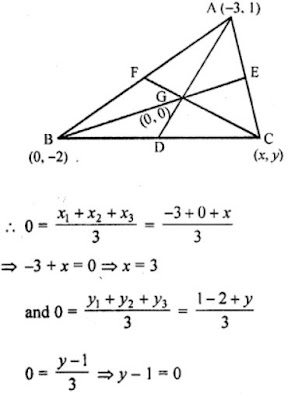∴ y = 1
∴ Coordinates of third vertex C are (3, 1)

4. A (3, 2) and B (-2, 1) are two vertices of a triangle ABC whose centroid G has the coordinates (5/3, -1/3) . Find the coordinates of the third vertex C of the triangle.

Solution

A (3, 2) and B (-2, 1) are the two vertices of ∆ABC whose centroid is G (5/3, -1/3)
Let third vertex C be (x, y)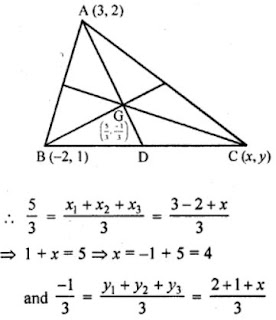⇒ 3 + x = -1
⇒ x = -1 - 3 = -4
∴ Coordinates of C will be (4, -4)

5. If (-2, 3), (4, -3) and (4, 5) are the mid-points of the sides of a triangle, find the co-ordinates of its centroid.

Solution

In ∆ABC, D, E and F are the mid-points of the sides BC, CA and AB respectively.
The co-ordinates of D are (-2, 3), of E are (4,-3) and of F are (4, 5)
Let the co-ordinates of A, B and C be (x1, y1), (x2, y2), (x3, y3) respectively.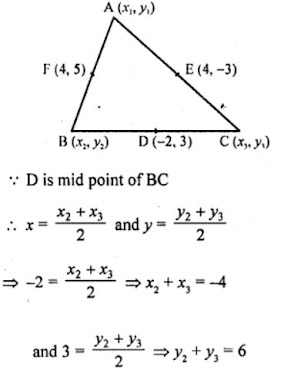Similarly, E and F are the mid points of AC and AB respectively.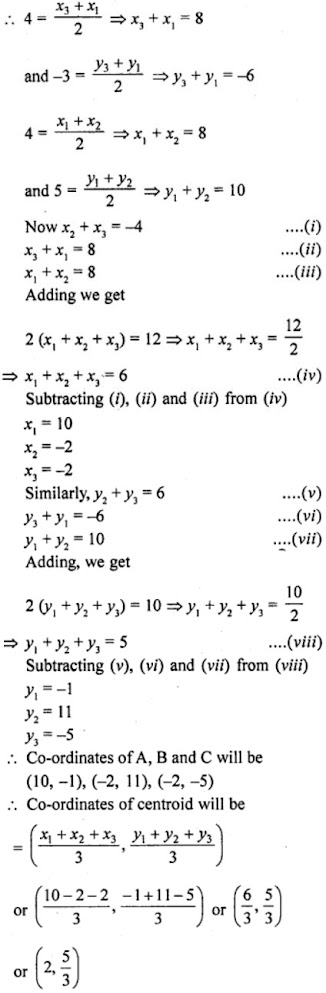6. Prove analytically that the line segment joining the middle points of two sides of a triangle is equal to half of the third side.

Solution

In ∆ABC,
D and E are the mid points of the sides AB and AC respectively.7. Prove that the lines joining the middle points of the opposite sides of a quadrilateral and the join of the middle points of its diagonals meet in a point and bisect one another.

Solution

Let A (x1, y1), B (x2, y2), C (x3, y3) and D (x4, y4) be the vertices of quadrilateral ABCD
E and F are the mid points of side BC and AD respectively and EF is joined G and H are the mid points of diagonal AC and BD.
GH are joined.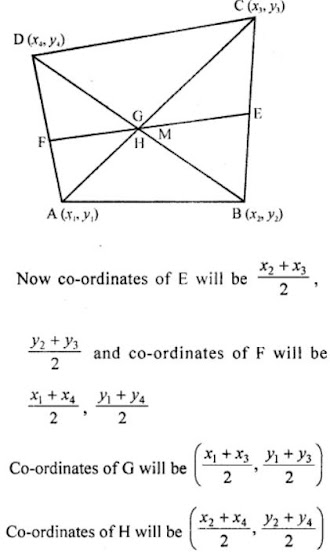EF and Gh intersect each other at M.
Let M is the mid-point of EF, then its coordinates will be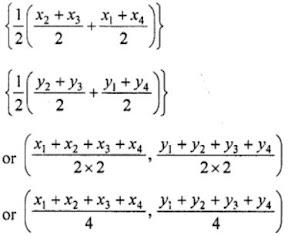Let M is the mid-point of GH, then its coordinates of M will be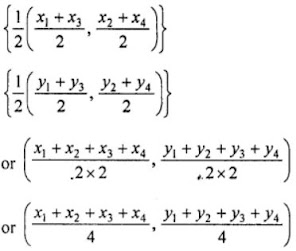We see the coordinates of in both cases are same.
EF and GH bisect each other at M.
Hence, proved.

8. If G be the centroid of a triangle ABC and P be any other point in the plane, prove that PA² + PB² + PC² = GA² + GB² + GC² + 3GP².

Solution

In AABC, G is the centroid of it Let P (h, x) is any point in the plane
Let co-ordinates of A are (x1, y1) of B are (x2, y2) and of C are (x3, y3)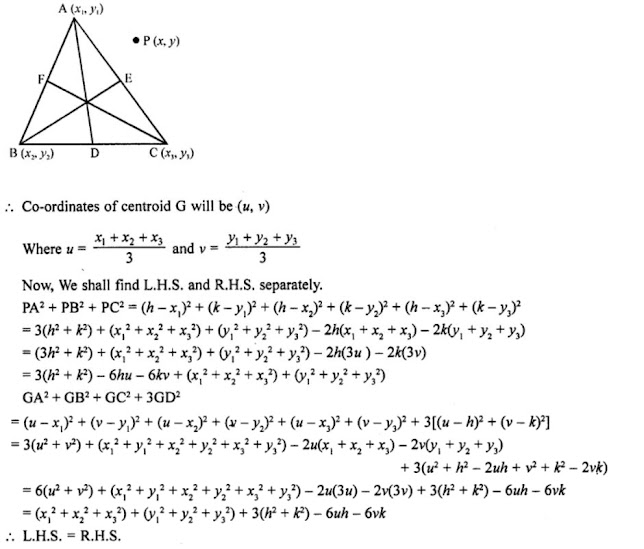9. If G be the centroid of a triangle ABC, prove that AB² + BC² + CA² = 3 (GA² + GB² + GC²)

Solution

Let the co-ordinates of the vertices of ∆ABC be A (x1, y1), B (x2, y2), C (x3, y3) and let G be the centroid of the triangle.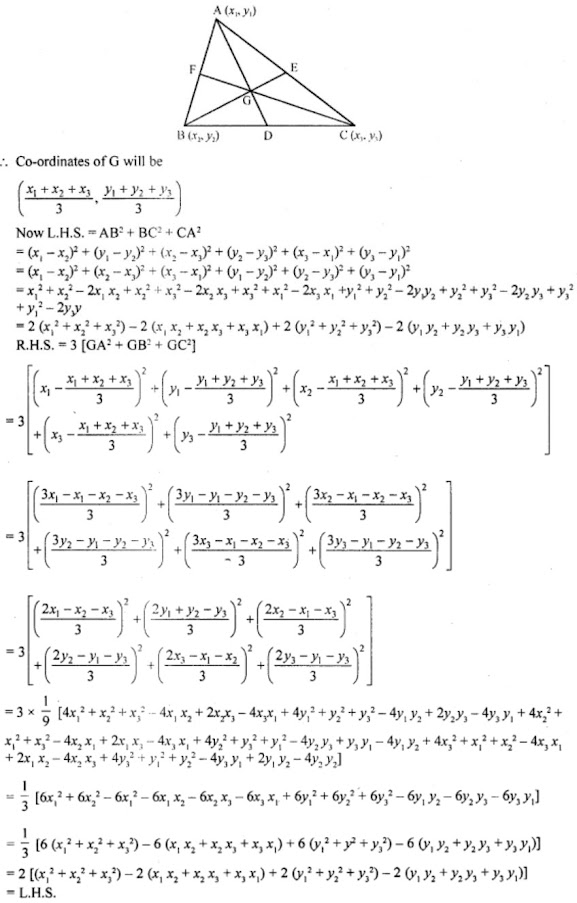Hence, proved.

10. In the figure, a right triangle BOA is given. C is the mid-point of the hypotenuse AB. Show that it is equidistant from the vertices O, A and B.

Solution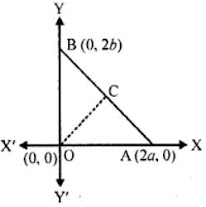In right ∆OAB, co-ordinates of O are (0, 0) of A are (2a, 0) and of B are (0, 2b)
C is the mid-point of AB
Co-ordinates of C will be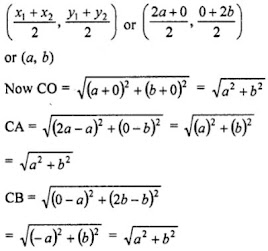We see that CO = CA = CB
Hence, C is equidistant from the vertices O, A and B.
Hence, proved.